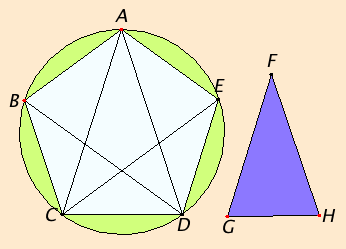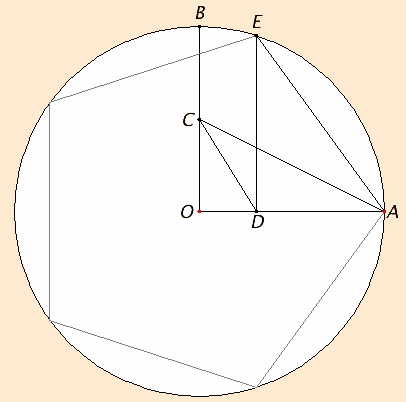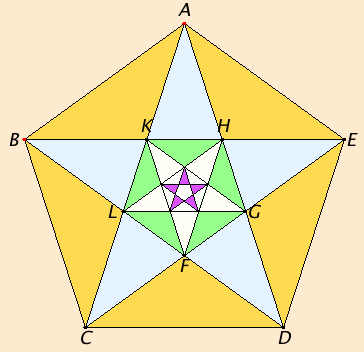# Proposition 11

To inscribe an equilateral and equiangular pentagon in a given circle.

Let ABCDE be the given circle.

It is required to inscribe an equilateral and equiangular pentagon in the circle ABCDE.

IV.10

Set out the isosceles triangle FGH having each of the angles at G and H double the angle at F.

IV.2

Inscribe in the circle ABCDE the triangle ACD equiangular with the triangle FGH, so that the angles CAD, ACD, and CDA equal the angles at F, G, and H respectively. Therefore each of the angles ACD and CDA is also double the angle CAD.I.9

Now bisect the angles ACD and CDA respectively by the straight lines CE and DB, and join AB, BC, DE, and EA.

Then, since each of the angles ACD and CDA is double the angle CAD, and they are bisected by the straight lines CE and DB, therefore the five angles DAC, ACE, ECD, CDB, and BDA equal one another.

III.26

But equal angles stand on equal circumferences, therefore the five circumferences AB, BC, CD, DE, and EA equal one another.

III.29

But straight lines that cut off equal circumferences are equal, therefore the five straight lines AB, BC, CD, DE, and EA equal one another. Therefore the pentagon ABCDE is equilateral.

I say next that it is also equiangular.

For, since the circumference AB equals the circumference DE, add BCD to each, therefore the whole circumference ABCD equals the whole circumference EDCB.

III.27

And the angle AED stands on the circumference ABCD, and the angle BAE on the circumference EDCB, therefore the angle BAE also equals the angle AED.

For the same reason each of the angles ABC, BCD, and CDE also equals each of the angles BAE and AED, therefore the pentagon ABCDE is equiangular.

But it was also proved equilateral, therefore an equilateral and equiangular pentagon has been inscribed in the given circle.

Q.E.F.

## Guide

#### Richmond’s construction

 The construction of this proposition is rather tedious to carry out. First, a line has to be cut according to the construction in II.11. Next, that is used in IV.10 for the construction of a 36°-72°-72° isosceles triangle. Next, that triangle is fit into the given circle using the construction IV.2. Finally, a couple more lines are drawn to finish the pentagon. Various alternatives have have been given by others, such as Ptolemy. One of the nicest was given in 1893 by H. W. Richmond. To inscribe a regular pentagon in a circle, first draw perpendicular radii OA and OB from the center O of a circle. Let C be the midpoint of OB and draw AC. Bisect angle ACO to meet OA at D. Draw a perpendicular DE to OA to the circle. Then AE is one side of the pentagon. The remaining sides can then be constructed. The easiest way to verify that Richmond’s construction works is by means of trigonometry.#### The angles and sections in extreme and mean ratio in a pentagram

Consider the regular pentagon ABCDE constructed in this proposition.Draw the diagonals of the pentagram to create a regular star pentagram ACEBD inside the pentagon. These diagonals meet forming a smaller regular pentagon in the center of the original pentagon. The diagonals of that pentagon can be drawn to make an inscribed pentagram which, in turn, bound a yet smaller regular pentagon. And so forth. For purposes of analysis, let d1 and s1 denote the diagonal and side of the first regular pentagon ABCDE. Also let d2 and s2 denote the diagonal and side of the second regular pentagon FGHKL. And so forth. It is evident that there are many lines parallel to the base CD of the triangle, namely BE and LG, as well as innumerable ones in the smaller pentagrams. That means that there will be many 36°-72°-72° triangles besides the large one ACD. The next smaller one is ALG, then FKH, then many smaller ones. Also, each of these various sized 36°-72°-72° triangles is congruent many others in the diagram. For instance, triangles ALG and EAK are congruent.

There is also a series of obtuse 36°-36°-108° isosceles triangles of varying sizes.

All these parallel lines and similar triangles yield numerous relationships among the various diagonals and sides of the pentagons. Some of these relationships are additive equations:

d1 = s1 + d2
s1 = d2 + s2
d2 = s2 + d3
s2 = d3 + s3
and so forth.

Other relationships are based on the property of 36°-72°-72° triangles used in their construction in IV.10, namely that the square of the base of such a triangle equals the product of a side and the difference between the side and the base. In terms of the diagonals and sides of the pentagons, this gives the equations:

d1 d2 = s12
s1 s2 = d22
d2 d3 = s22
s2 s3 = d32
and so forth.
After ratios are proportions are developed in Book V and Book VI, we can add the following continued proportion to the list of relationships:
d1:s1 = s1:d2 = d2:s2 = s2:d3 = ...
See the Guide to proposition X.2 which shows that diagonal and side of a regular pentagon are incommensurable. In more modern terms we would say that their ratio, which is called the “golden ratio,” is an irrational number.

#### Use of this proposition

This construction is used in the next proposition to circumscribe a regular pentagon around a circle and later in IV.16 to construct a regular 15-gon. It is also used in XIII.16 for the construction of a regular icosahedron (a 20-sided polyhedron each of whose faces is an equilateral triangle). Surprisingly, it is not used in XIII.17 for construct a regular dodecahedron (a 12-sided polyhedron each of whose faces is a regular pentagon); the regular pentagons needed for it are constructed in space directly without the help of this proposition.